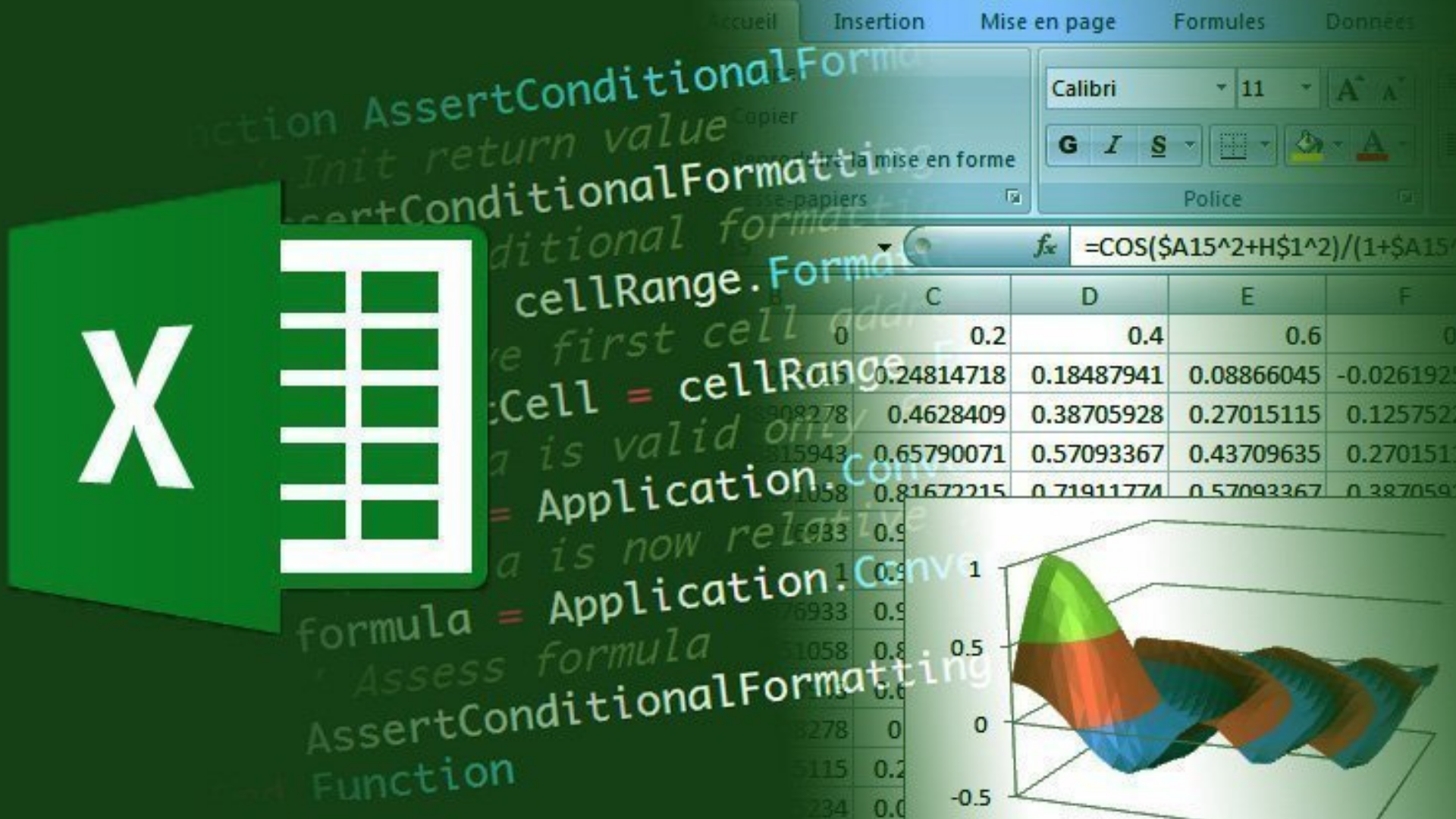# FAQ About Excel Visual Basic for Applications

## What is Excel VBA?

It is an adapted version of the Visual Basic language for use in Microsoft Office programs.

## How to enable Excel's Developer section?

First, click on File in the upper left. Afterwards, the Options at the bottom left of the page should be selected.

A pop-up options page will appear. Here we choose the Customize Ribbon option from the options on the left.

We make it enabled by clicking the Developer option from the options on the right. When you click to Okay, you will see the Developer section on the top menu in Excel.

## How to reach coding interface in Excel?

There are two options here;

• By clicking the Visual Basic button on the left, the first one is under the top menu,
• Or it can be viewed with the View Code button on the right of the same menu.

In addition, when right-clicking on the name of the page below and selecting View Code, it works as in the second option.

## Why is code written in a module in Excel?

If you want to create a function, code must be written in the module. Or, if there is a reference to this code in the relevant Excel library, the code should be written in the module.

## What is the difference between Form Controls and ActiveX Controls on the Developer tab?

Form Controls are built into Excel, while ActiveX Controls are installed separately.

For example, one of the simple differences can be explained through the List Box.

When a List Box is created with Form Controls, it takes the index value of those values in the cell where the values in the List Box will go. However, when a List Box is created with ActiveX Controls, these values appear directly in the cell.

## What are the data types in Excel VBA?

Data types in Excel are divided as numeric and non-numeric types.

Numeric data types;

• Integer
• Double
• Long
• Bytes
• Single
• Currency
• Decimal

Non-numeric data types;

• String
• Boolean
• Date
• Object
• Variant
• User-defined

## How to create a variable in code panel in Excel?

First we use the Dim command from Dimension to create a variable.

Then we write the name we want to give to the variable.

Then we type As and specify the type of the variable. We write one of the types like string, integer etc.

The result should look like this;

• Dim variableName As Integer

## How do we assign values to variables in Excel?

It's as easy as specifying with an equal sign. For example, let's say you are creating a numeric variable.

• Dim numericVariable As Integer

Let's say you want to give the value 10 to this variable from here on out. When you type it to the code panel this;

• numericVariable = 10

the value 10 will be assigned to your variable.

## Where can I see the results of my codes that I wrote in Excel?

We can see the results of the codes with a message box or we can send them to a cell.

The code you need to write to display it with the message box;

Result: The name of the variable with the result value.

• MsgBox result

When you run this code, you will see a message box pop-up with the result.

But let's say you want to display the result in cell A1. Then the code you need to write;

• Range("A1") = result

When you run the code, the result will be written in cell A1.

## Can we assign a value in a cell to a variable in Excel?

Yes, we can.

For example, let's say you have a value in cell B1. The code you need to write to assign this value to your variable;

• variableName = Range("B1")